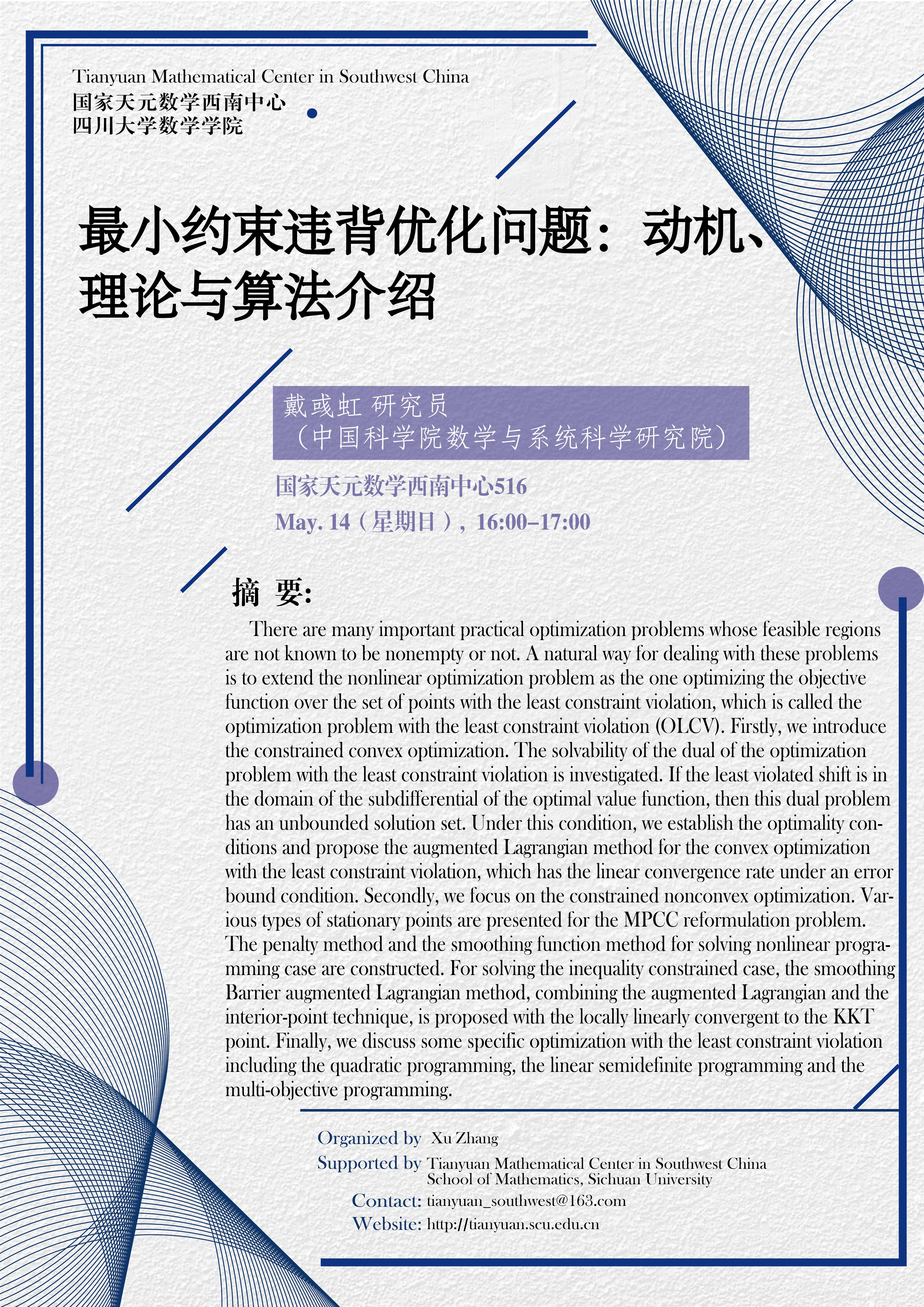There are many important practical optimization problems whose feasible regions are not known to be nonempty or not. A natural way for dealing with these problems is to extend the nonlinear optimization problem as the one optimizing the objective function over the set of points with the least constraint violation, which is called the optimization problem with the least constraint violation (OLCV). Firstly, we introduce the constrained convex optimization. The solvability of the dual of the optimization problem with the least constraint violation is investigated. If the least violated shift is in the domain of the subdifferential of the optimal value function, then this dual problem has an unbounded solution set. Under this condition, we establish the optimality conditions and propose the augmented Lagrangian method for the convex optimization with the least constraint violation, which has the linear convergence rate under an error bound condition. Secondly, we focus on the constrained nonconvex optimization. Various types of stationary points are presented for the MPCC reformulation problem. The penalty method and the smoothing function method for solving nonlinear programming case are constructed. For solving the inequality constrained case, the smoothing Barrier augmented Lagrangian method, combining the augmented Lagrangian and the interior-point technique, is proposed with the locally linearly convergent to the KKT point. Finally, we discuss some specific optimization with the least constraint violation including the quadratic programming, the linear semidefinite programming and the multi-objective programming.